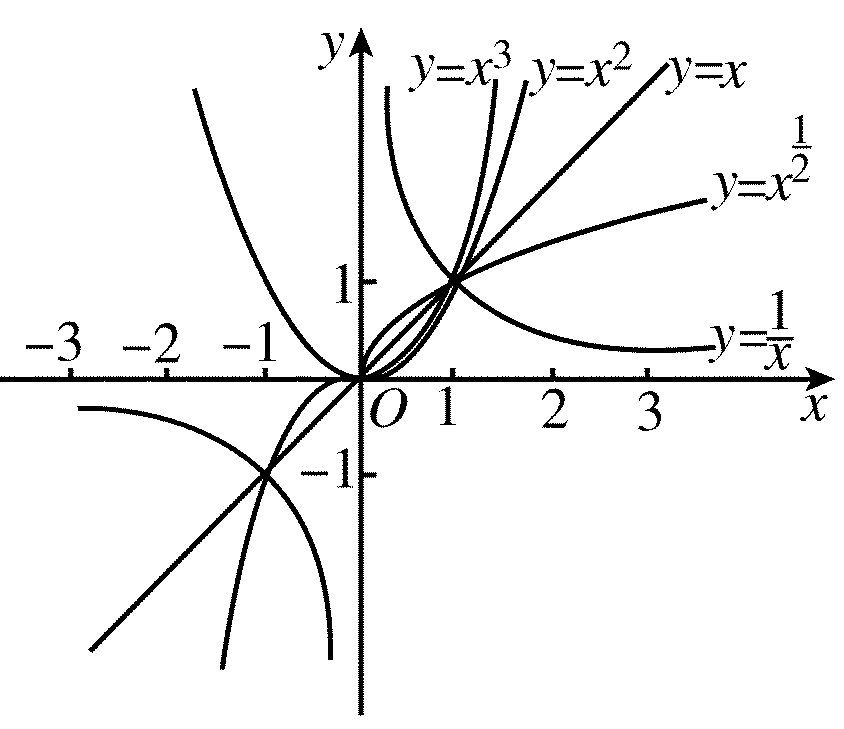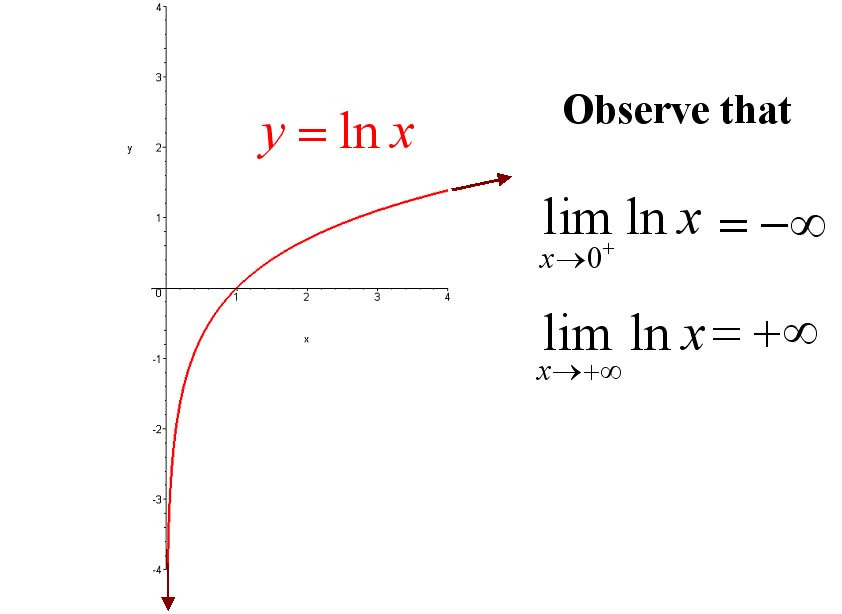## 高等数学计划·0312 📖：

#### 函数和映射（基本初等函数）：

##### 甩图：##### 课后习题：

（1）

$\begin{array}{l}y=\tan x, x \neq\left(k+\frac{1}{2}\right) \pi, k \in \mathbf{Z} \\ y=\cot x, x \neq k \pi, k \in \mathbf{Z} \\ y=\arcsin x,|x| \leqslant 1 \\ y=\arccos x,|x| \leqslant 1\end{array}$

\begin{aligned} y &=\frac{Q(x)}{P(x)}, P(x) \neq 0 \\ y &=\sqrt[2 n]{x}, x \geqslant 0 \\ y &=\log _{a} x, x>0 \end{aligned}

$y＝arcsin(x)，domain：[-1,1] ，range：[-π/2,π/2]\\y=arccos(x)domain：[-1,1] ，range：[0,π] \\y=arctan(x)domain(-∞,+∞)，range：(-π/2,π/2) \\y=sinarcsin(x)=x,domain：[-1,1],range：[-π/2,π/2]$

2.求解以下函数单调性，区间固定。

$\begin{array}{llll}\text { (1) } y=\frac{x}{1-x}, & (-\infty, 1) ; & \text { (2) } y=x+\ln x, & (0,+\infty)\end{array}$

（1）单调递增：$\ let \ x_2>x_1 \ y_2 - y_1 = \frac{x_{2}-x_{1}}{\left(1-x_{1}\right)\left(1-x_{2}\right)}\\1-x_1>0 \ and \ 1-x_2 >0 \ \to y_2 -y_1 >0$

（2）$x_1-x_2 >0 \\ ln \ image \ get \ \ln(x_2)0$

5.奇函数定义可得

11.复合函数求解，不再赘述。